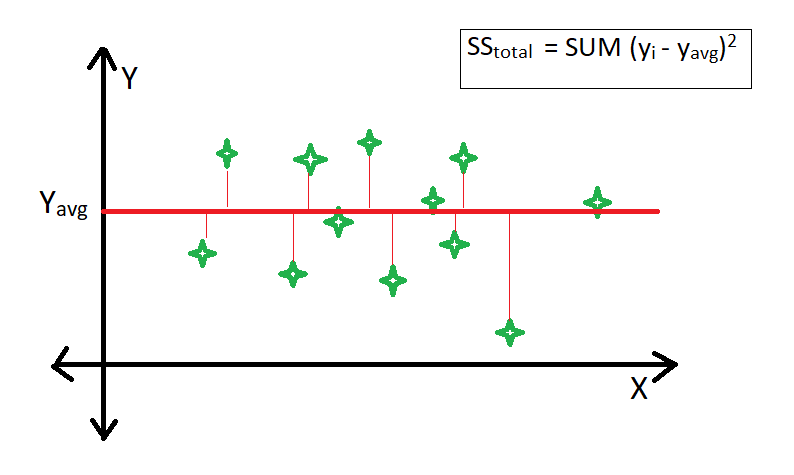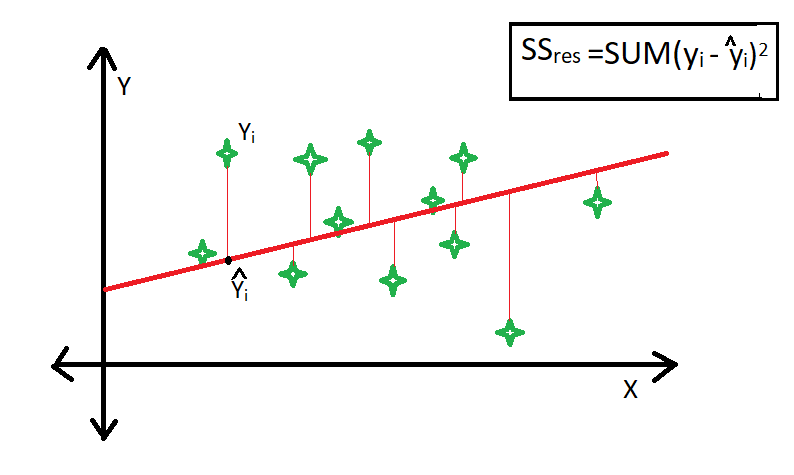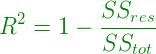Related Articles
ML | R-squared in Regression Analysis
• Last Updated : 07 May, 2019

R-squared is a statistical measure that represents the goodness of fit of a regression model. The ideal value for r-square is 1. The closer the value of r-square to 1, the better is the model fitted.

R-square is a comparison of residual sum of squares (SSres) with total sum of squares(SStot). Total sum of squares is calculated by summation of squares of perpendicular distance between data points and the average line.Residual sum of squares in calculated by the summation of squares of perpendicular distance between data points and the best fitted line.R square is calculated by using the following formula :Where SSres is the residual sum of squares and SStot is the total sum of squares.

The goodness of fit of regression models can be analyzed on the basis of R-square method. The more the value of r-square near to 1, the better is the model.

Note : The value of R-square can also be negative when the models fitted is worse than the average fitted model.

Limitation of using R-square method –

• The value of r-square always increases or remains same as new variables are added to the model, without detecting the significance of this newly added variable (i.e value of r-square never decreases on addition of new attributes to the model). As a result, non-significant attributes can also be added to the model with an increase in r-square value.
• This is because SStot is always constant and regression model tries to decrease the value of SSres by finding some correlation with this new attribute and hence the overall value of r-square increases, which can lead to a poor regression model.My Personal Notes arrow_drop_up